## 概率统计14——几何分布

我家小朋友年方1岁半，家里每天上午都要出去遛小孩。现在小朋友有两项爱好，在家翻垃圾桶，出门捡烟头。

翻垃圾桶可以有效地限制，捡烟头可是防不胜防。

也许烟头能散发出特殊的能量波动，小区的绿化带和草坪上的大部分烟头都能被小朋友准确地发现，如果他在不规则的前进路线中突然停下了，那肯定是看到了新的烟头。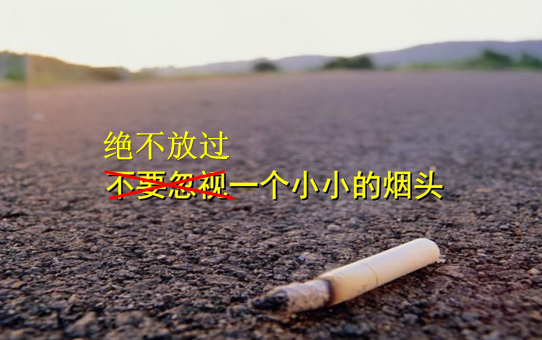# 错失奖励概率

在我的严密监视下，小朋友捡烟头的几率已经从原来的“绝不放过”下降到了现在的20%，如果他直到回家还没有烟头，就能得到一个棒棒糖作为奖励。当然，每个烟头对他来说仍然存在着独特的魅力，在最初遇到的3个烟头中没有经受住考验的概率是多少？

设随机变量X是小朋友最终捡起烟头时所发现的烟头个数，那么：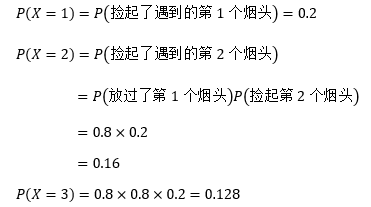最初遇到的3个烟头中没有抵抗住诱惑的概率可以用P(X ≤ 3)表示：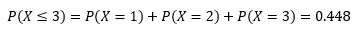每个烟头都是一次考验，我的关注点是，小朋友在第几次考验时会捡起烟头？

# 质量函数

小朋友每次遇到烟头的行为都是一个独立的随机试验，试验只有成功和失败两种结果，且对于每个随机试验来说，成功的概率都是相同的。对于小朋友自己来说，捡起烟头是成功，错过才是失败，只要成功一次就没有棒棒糖，试验结束。现在用p表示成功率（捡起烟头的概率），q = 1 – p表示失败率，随机变量X表示第一次成功时所经历的试验次数，那么在进行了r次试验后才遇到第一次成功的概率（或者说在第r次试验取得成功前，需要经历r-1次失败的概率）可以表示为：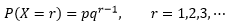这个分布就是几何分布（Geometric distribution），X服从几何分布，记为X～GE(p)。

下面的python代码展示了r取不同值时的P(X=r)。

 1 import numpy as np
2 import matplotlib.pyplot as plt
3 from scipy import stats
4
5 p = 0.2 # 成功率
6 print('X ~ GE({0})'.format(p))
7
8 rs = np.array(range(1, 11, 1)) # 随机变量的取值
9 # 几何分布 X ~ GE(0.2)
10 ps = stats.geom.pmf(rs, p) # 每个随机变量对应的概率
11 for i, r in enumerate(rs):
12     print('P(X={0})={1}'.format(r, ps[i]))
13
14 plt.bar(left=rs, height=ps, width=0.5)
15 plt.xlabel('r, X=r表示第r次试验才成功')
16 plt.ylabel('P(X=r)')
17 plt.rcParams['font.sans-serif'] = ['SimHei']  # 用来正常显示中文标签
18 plt.show()

X ~ GE(0.2)

P(X=1)=0.2

P(X=2)=0.16000000000000003

P(X=3)=0.12800000000000003

P(X=4)=0.10240000000000003

P(X=5)=0.08192000000000002

P(X=6)=0.06553600000000002

P(X=7)=0.052428800000000025

P(X=8)=0.041943040000000015

P(X=9)=0.033554432000000016

P(X=10)=0.026843545600000015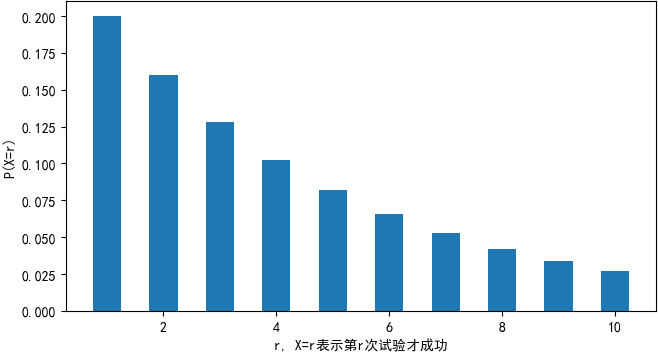对于几何分布来说，r是大于等于1的自然数，并且只有成功的概率处于(0, 1)之间才有意义，因此当r = 1时，P(X = r)达到最大值，随着r的增大，P(X = r)也越来越小。

几何分布是一个典型的长尾分布，第一次就成功的概率是最高的，这看似有违直觉。这里需要注意的是，“第r次才取得成功”和“在r次之内取得成功”是两回事，后者才是概率越来越大。

实际中有不少随机变量服从几何分布，例如某产品的不合格率为0.05，则首次查到不合格品的检查次数X~GE(0.05)。

# 分布的形状

现在回到问题的关注点：小朋友在第几次考验时会捡起烟头？这个问题并不能确切地回答，可以回答的是，在r次考验之内捡起烟头的概率。这个概率可以用分布函数表示：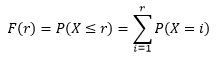另一种计算方法是：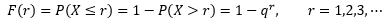P(X > r)表示在取得第一次成功时，前r次试验都失败的概率。F(r)更为专业的说法是：为得到1次成功而进行r次伯努利试验，r的概率分布。

下面的代码展示了几何分布的形状：

1 fs = stats.geom.cdf(rs, p) # 每个r对应的分布
2 for i, r in enumerate(rs):
3     print('F({0})=P(X<={0})={1}'.format(r, fs[i]))
4
5 plt.bar(left=rs, height=fs, width=0.5)
6 plt.xlabel('r, X<=r表示前r次试验至少有一次成功')
7 plt.ylabel('F(r) = P(X <= r)')
8 plt.rcParams['font.sans-serif'] = ['SimHei']  # 用来正常显示中文标签
9 plt.show()

F(1)=P(X<=1)=0.19999999999999998)

F(2)=P(X<=2)=0.36)

F(3)=P(X<=3)=0.488)

F(4)=P(X<=4)=0.5904)

F(5)=P(X<=5)=0.67232)

F(6)=P(X<=6)=0.7378560000000001)

F(7)=P(X<=7)=0.7902848)

F(8)=P(X<=8)=0.83222784)

F(9)=P(X<=9)=0.865782272)

F(10)=P(X<=10)=0.8926258176)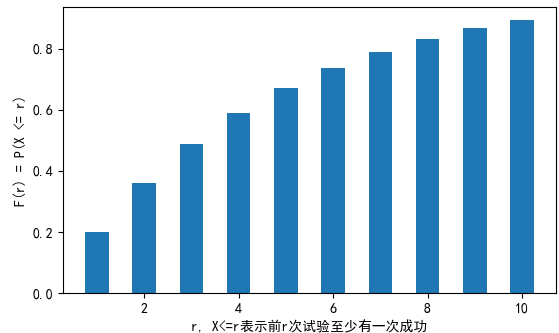小朋友大约有89%的概率在10次考验内捡起烟头，他也因此很少得到棒棒糖。

# 期望和方差

X ~ GE(p)，q=1-p，P(X = r) = pqr-1，当r→∞时：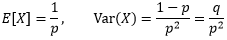来看看是怎么得出的。

## 期望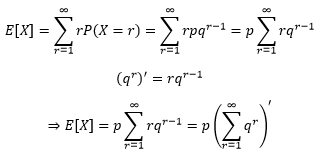可以根据几何级数的公式继续计算：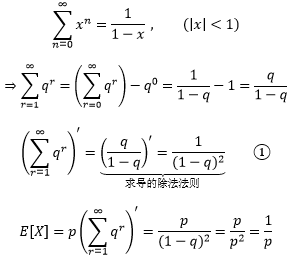对于几何分布来说，p=0.2表示单次试验成功的概率是0.2，E[X]=1/p=5是在告诉我们，期望在第5次试验时获得成功，或者说5次试验中就有一次趋向成功。

## 方差

根据Var(X) = E[X2] – E[X]2来计算几何分布的方差：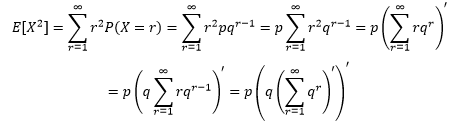将①代入：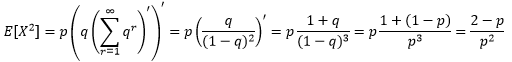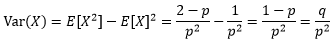出处：微信公众号 "我是8位的"

本文以学习、研究和分享为主，如需转载，请联系本人，标明作者和出处，非商业用途！

扫描二维码关注作者公众号“我是8位的”posted on 2020-01-17 16:16  我是8位的  阅读(950)  评论(0编辑  收藏